# Find the general solution of the following system of differential Equations. Show all the steps of...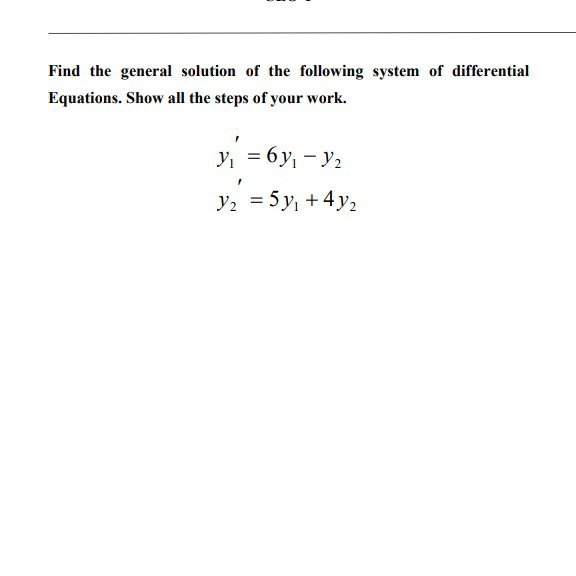Find the general solution of the following system of differential Equations. Show all the steps of your work. y = 6y; - y2 y2 = 5 y, +4y2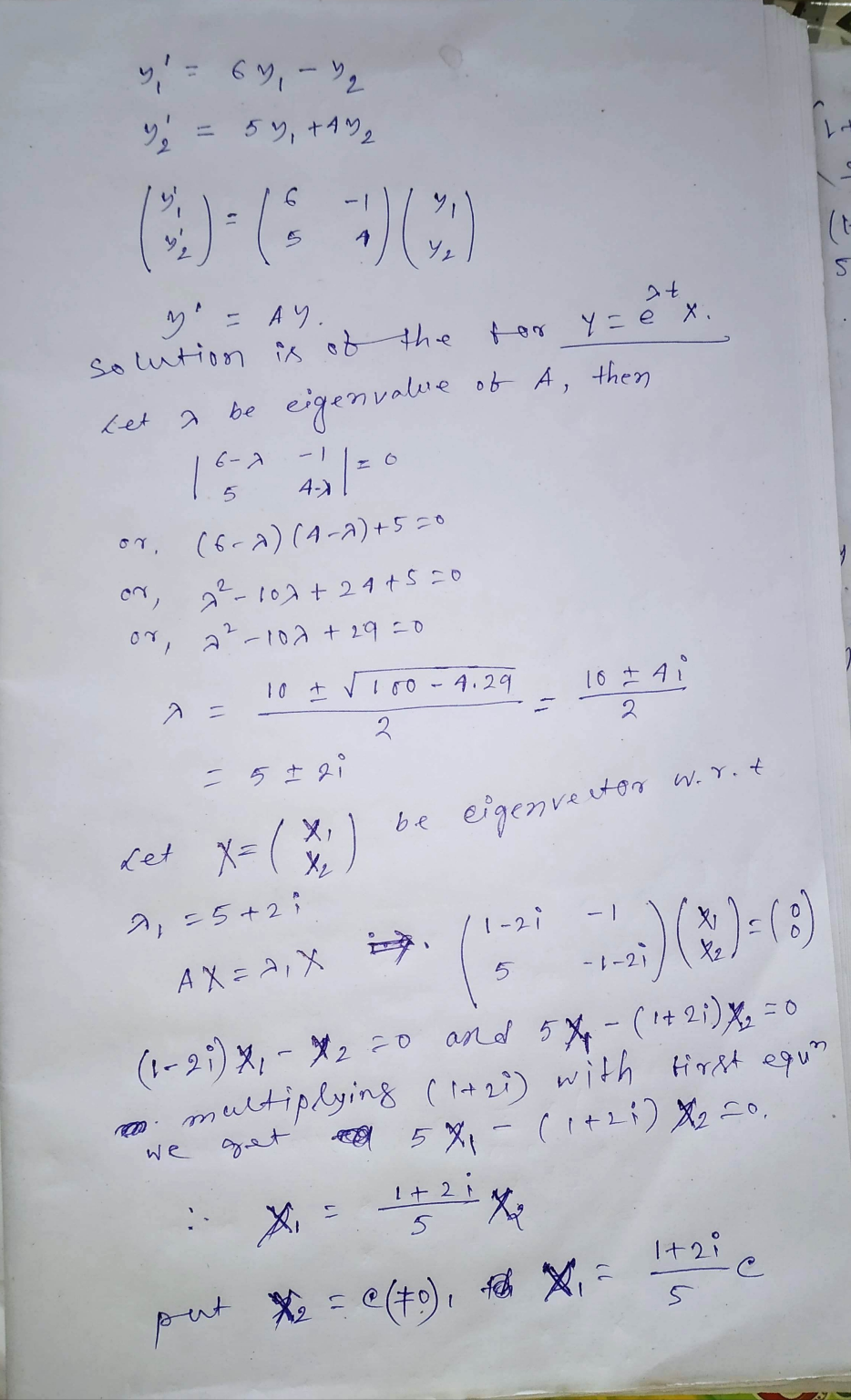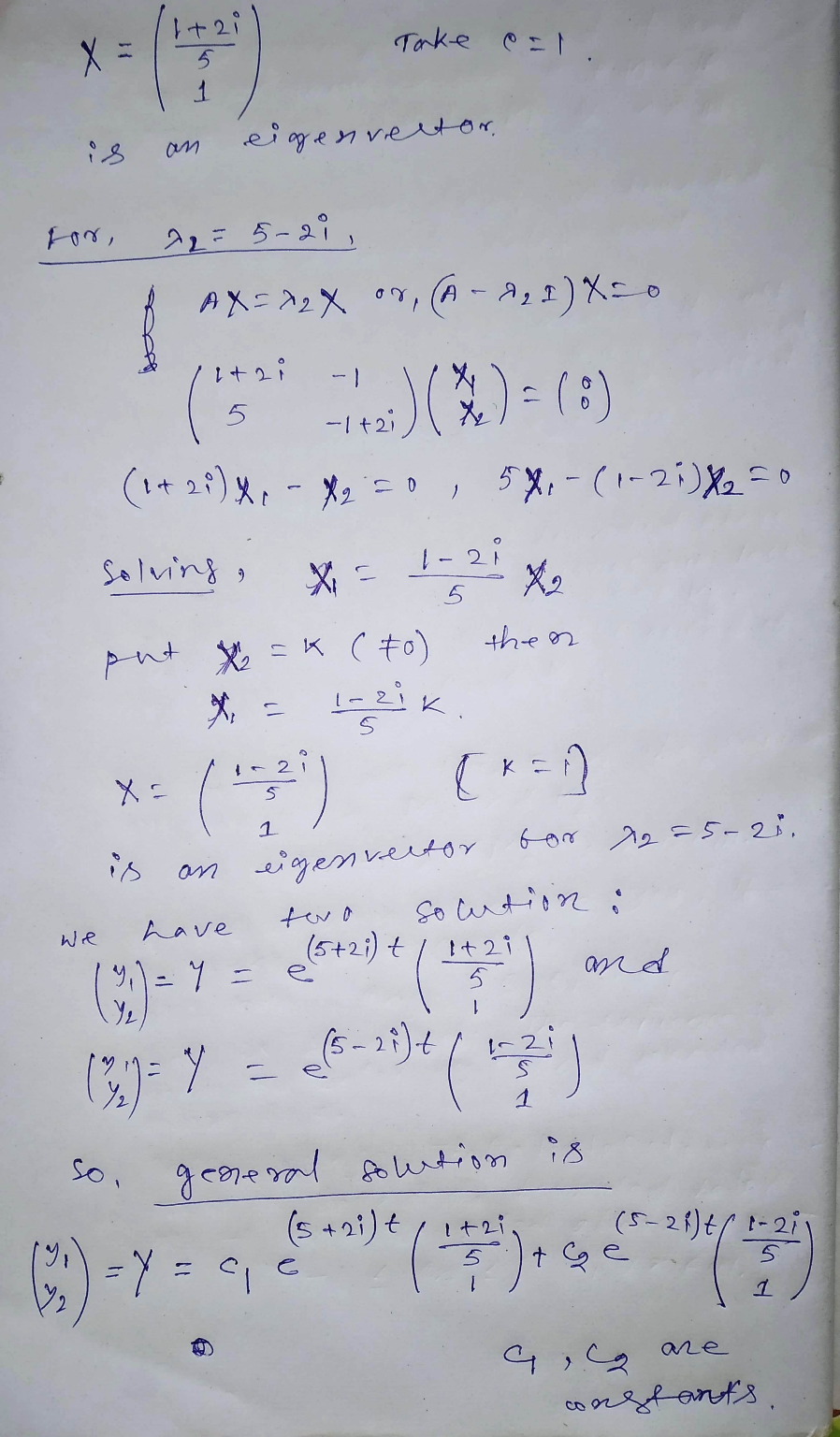##### Add Answer of: Find the general solution of the following system of differential Equations. Show all the steps of...
Similar Homework Help Questions
• ### Solve the Differential problem? Find the general solution of the following system of differential Equations. Show...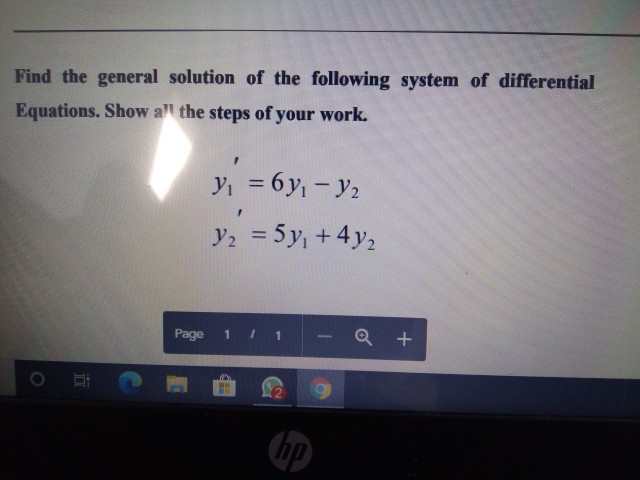Solve the Differential problem? Find the general solution of the following system of differential Equations. Show a" the steps of your work. y = 6 yı - y2 V2 = 5y +442 Page 1 a + (hp)

• ### please answer all the following quesitons and show all working 1. Find the general solution to...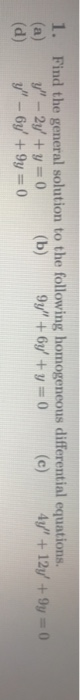please answer all the following quesitons and show all working 1. Find the general solution to the following homogeneous differential equations. y" - 2y' + y = 0 (b) 9y" + 6y' + y = 0 4y' +12y +9y=0 (d) y" - 6y' +9y = 0

• ### Please show solutions. Answer: 1. Find a general solution to the following differential equations: (a) y"...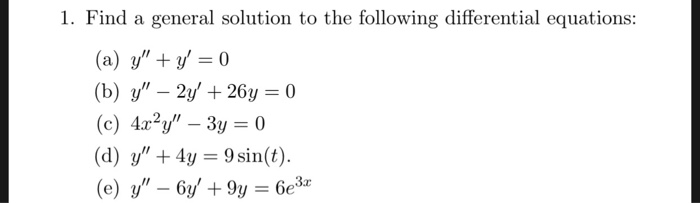Please show solutions. Answer: 1. Find a general solution to the following differential equations: (a) y" + y = 0 (b) y" – 2y' + 264 = 0 (c) 4x²y" – 3y = 0 (d) y" + 4y = 9 sin(t). (e) y" – 6y' + 9y = 6e3x 1. (a) y = ci + c2e- (b) y = cle' cos(5t) + czet sin(5t) (c) y = cit-1/2 + c2t3/2 (d) y = ci cos(2t) + c2 sin(2t) + 3...

• ### 1. Find the general solution for each of the following differential equations (10 points each): y"...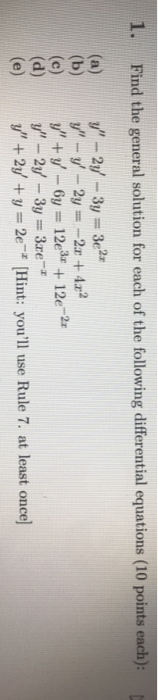1. Find the general solution for each of the following differential equations (10 points each): y" - 2y - 3y = 32 y" - y' - 2y = -2 + 4.2 y" + y' - 6y = 12e3+ + 12e-2x y" - 2y - 3y = 3.re* y" + 2y + y = 2e-* (Hint: you'll use Rule 7. at least once)

• ### 3. Consider the first-order system of differential equations: (a) Find the general real-valued solutions (b) Find...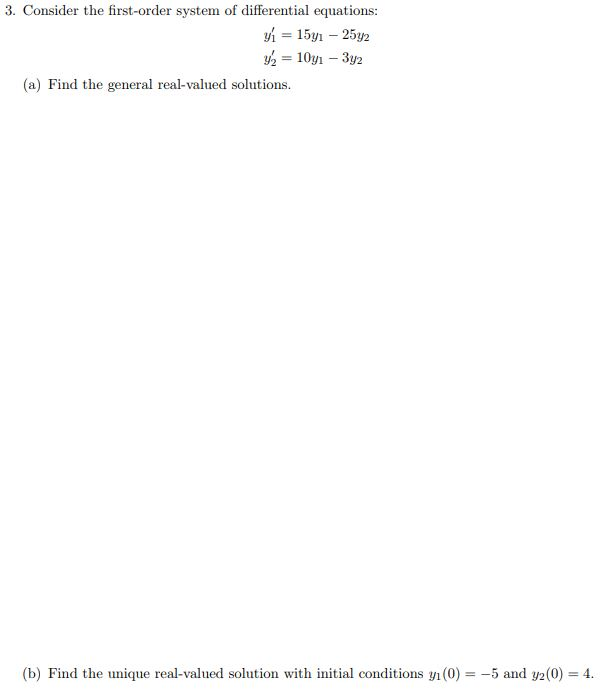3. Consider the first-order system of differential equations: (a) Find the general real-valued solutions (b) Find the unique real-valued solution with initial conditions yi (0) = 5 and y2(0) = 4.

• ### this is from differential equations ch8 section 2. please write clearly and show all steps Use...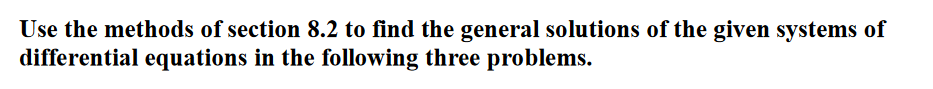this is from differential equations ch8 section 2. please write clearly and show all steps Use the methods of section 8.2 to find the general solutions of the given systems of differential equations in the following three problems. 2. dx dt 2x + y dy = -x + 4y dt

• ### Find the general solution of the following differential equation: (1) ?′′ + 5?′ + 6? =...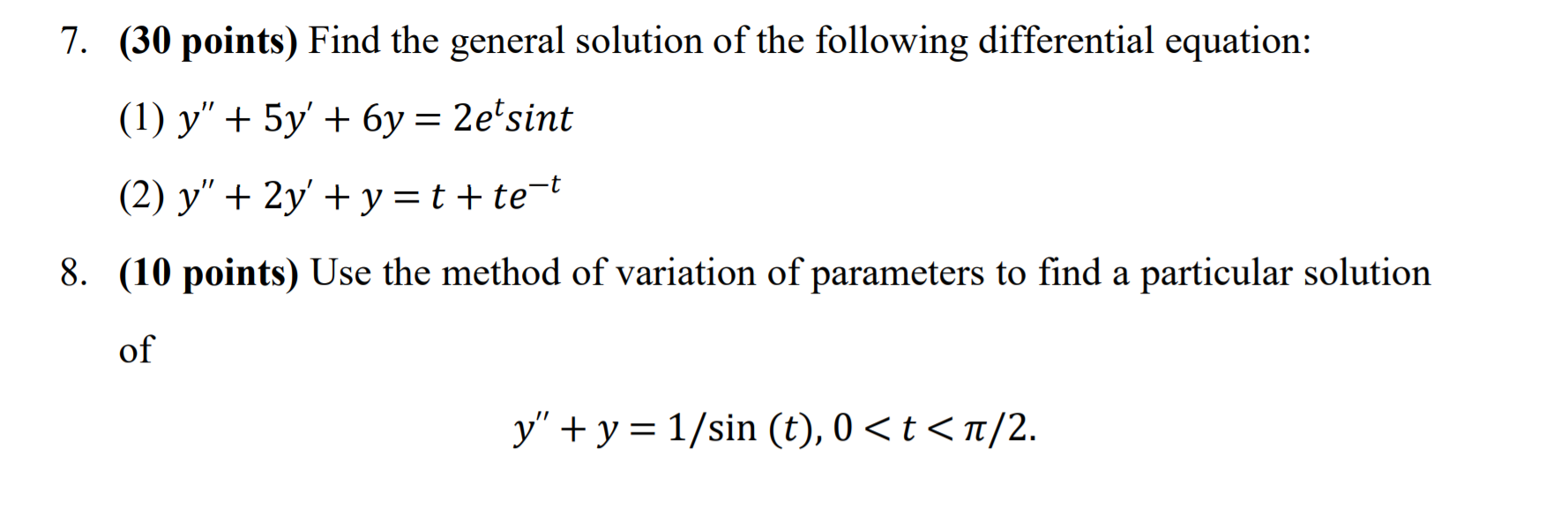Find the general solution of the following differential equation: (1) ?′′ + 5?′ + 6? = 2????*?^? (2) ?′′ + 2?′ + ? = ? + ?e^(-t). (please solve Question No.7 only) 7. (30 points) Find the general solution of the following differential equation: (1) y" + 5y' + 6y = 2etsint (2) y" + 2y + y=t+te-t 8. (10 points) Use the method of variation of parameters to find a particular solution of y" + y = 1/sin (t),...

• ### 2. Differential equations and direction fields (a) Find the general solution to the differential equation y'...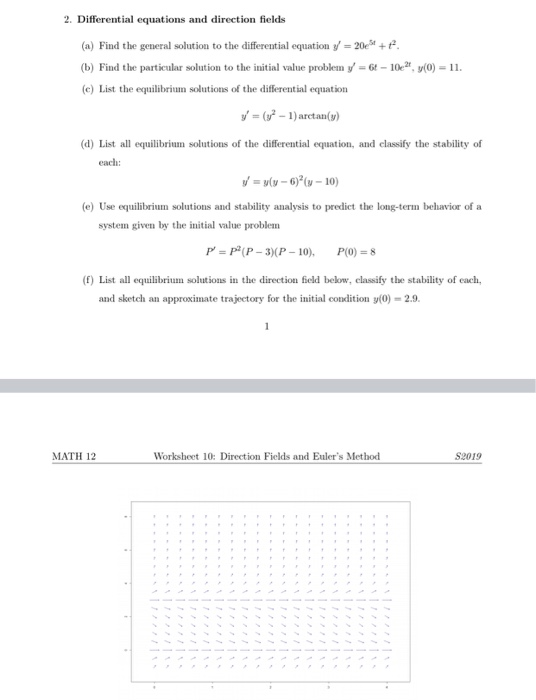2. Differential equations and direction fields (a) Find the general solution to the differential equation y' = 20e3+ + + (b) Find the particular solution to the initial value problem y' = 64 – 102, y(0) = 11. (e) List the equilibrium solutions of the differential equation V = (y2 - 1) arctan() (d) List all equilibrium solutions of the differential equation, and classify the stability of each: V = y(y - 6)(n-10) (e) Use equilibrium solutions and stability analysis...

• ### differential equations-system of Differential Equations-Complex Egienvvalues

Complex Eidenvalues(A) convert the IVP into a system of differential equations and write your answer in the matrix form.(B) solve the system and use this solution to find the solution to the orginal 2ndorder IVP.(C) sketch the phase portrait for the systemadn determine the stability of the equilibrium solutiony''-6y'+58y=0y(0) =1,y'(0)= 0

• ### Find the general solution of y′′ + 2y = sin(7t/5) Please show all steps and work...

Find the general solution of y′′ + 2y = sin(7t/5) Please show all steps and work clearly so I can follow your logic and learn to solve similar ones myself. Will rate your answer. Thank you kindly!

Free Homework App